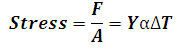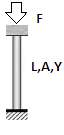# Thermal Stress of a fixed rod Calculator

This Calctown Calculator calculates the thermal stress in a fixed rod due to change in temperature.

Pa
K-1
K

#### Result

N/m2Click here to view image

where

F/A = Stress

Y = young's modulus

ΔT = change in temperature

α = coefficient of linear expansion